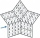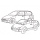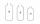# Divisibility + Pythagoras contest - math problems

#### Number of problems found: 5

• Five-digit numberAnna thinks of a five-digit number that is not divisible by three or four. If he increments each digit by one, it gets a five-digit number that is divisible by three. If he reduces each digit by one, he gets a five-digit number divisible by four. If it sw
• All pairsDetermine all pairs (m, n) of natural numbers for which is true: m s (n) = n s (m) = 70, where s (a) denotes the digit sum of the natural number a.
• Two cars on ringThere were two cars on the round track (ring) in the adjacent tracks, the first car in the inner track, the second in the outer track. Both cars started at the same time from one starting track. The first toy car drove every four laps simultaneously as th
• BicyclesYou're the owner of the transport 's learning playground. Buy bicycles of two colors but you've got to spend accurately 120000 Kč. Blue bike costs 3600Kč and red bicycle 3200Kč.
• FluidWe have vessels containing 7 liters, 5 liters and 2 liters. Largest container is filled with fluid the others empty. Can you only by pouring get 5 liters and two 1 liter of fluid? How many pouring is needed?

We apologize, but in this category are not a lot of examples.
Do you have an exciting math question or word problem that you can't solve? Ask a question or post a math problem, and we can try to solve it.

We will send a solution to your e-mail address. Solved examples are also published here. Please enter the e-mail correctly and check whether you don't have a full mailbox.

Divisibility - math problems. Pythagoras contest - math problems.